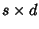## Criss-Cross Method

A standard form of the Linear Programming problem of maximizing a linear function over a Convex Polyhedron is to maximizesubject toand, where m is a givenmatrix,andare given-vector and-vectors, respectively. The Criss-cross method always finds a Vertex solution if an optimal solution exists.